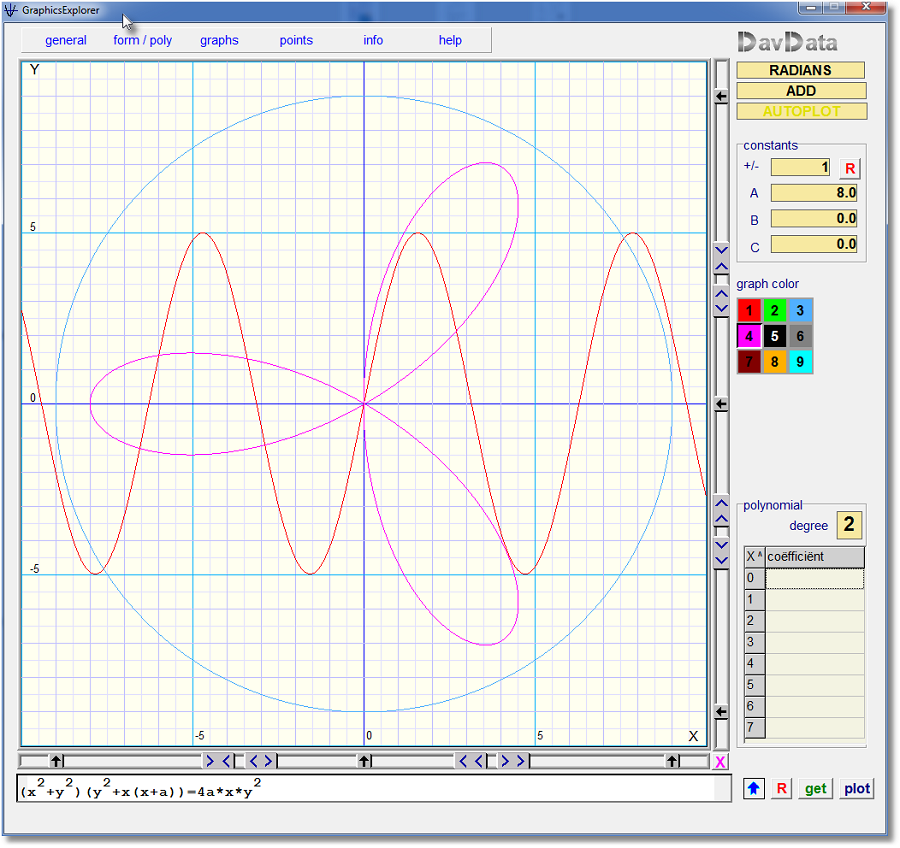Graphics Explorer Version 3download version 3.1download version 3.0

Version 3.1
- interim variable v for parametric functions is placed next to the coordinate system.

Version 3.0
- coordinate system of 800 x 800 pixels
- superscript formula editing (not x^2 but x2)
- formulas saved in .gxl file, together with color and settings

To see the (Delphi project) source code, look [ HERE ]

Below a reduced image (version 3.0):Installation

Graphics Explorer is written for Windows.
There is no installation procedure.
Just copy the .exe file to a folder of choice.

The program contains in-line help.

Features

- coordinate system 800 * 800 pixels
- crosshairs with (x,y) indication
- 9 different formulas or polynomials
- scrolling and zooming with mouseclick, x/y independent
- add or delete dots with mouseclick or by table editing
- calculate polynomial degree 0..7 through set of dots
- calculate exponential function through set of dots
- calculate circle through 3 dots
- recognizes 4 types of functions/equations:

typeexampledescription
1y = f(x)y = 3cos(5x)function
2x = f(y)x = (y-1)^2inverse
3y = f(v) ; x = g(v)y = 5sin(2v) ; x = 7cos(3v)parametric
4f(x) = g(x)(x2+y2)(y2+x(x+10))=40x*y2implicit

- enter polynomials, degree 0..7, in "coefficient" table
- operations on polynomials:
• reflections
• integration
• differentiation
• sum, difference, product
- use of constants a,b,c in formula's
- a,b,c changable by mouseclick, graphics adjust automatically
- plot function (type 1) 1..3 times differentiated
by simply preceding it by d:, dd: or ddd: .
example: y = d:sin(2x)
- plot function (type 1) integrated
by simply preceding it by i: .
example: y = i: 5sin(x)
- compute the area under a function
- exponential functions through set of dots:  y = a.bx y = a.bx + c y = a.ebx y = a.ebx + c y = eax^2 + bx + c
- all formula colors adjustable to personal preference.
Please report applications of graphxl so I can publish them.